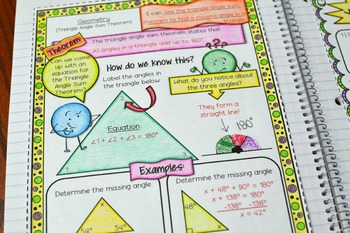# 8th Grade Math Interactive Notebook Great Tool as Study Guide & Very Organized7th - 9th
Subjects
Resource Type
Formats Included
• Zip
Pages
160 (280 including answer key)
##### Also included in
1. ⭐ This bundle includes ALL of my 8th Grade Math material in my store and ALL future 8th Grade Math Products ⭐This includes all of my popular 8th grade math interactive notebooks, practice worksheets, foldables, activities, warm-ups, homework, exit slips, assessments, early finishers, and SO MUCH MOR
Price \$249.99Original Price \$495.37Save \$245.38
2. Included in this bundle is my 6th, 7th, and 8th grade interactive notebooks. This is a great deal because I sell each notebook for \$59.99 individually. This means that you save over \$50 by purchasing this notebook bundle than buying them individually.The file is a zip file containing three PDF fil
Price \$143.98Original Price \$179.97Save \$35.99
3. ⭐ This bundle includes ALL of my Math Curriculum in my Teachers Pay Teachers store ⭐You will be receiving ALL of my 6th, 7th, and 8th Grade Math interactive notebooks, activities, foldables, practice worksheets, warm-ups, exit slips, assessments, and much more! This is well over 1000 pages of materi
Price \$899.99Original Price \$1718.65Save \$818.66
4. This is a custom bundle for E.S.Want a custom bundle? Email me at mathindemand@hotmail.com
Price \$115.18Original Price \$179.97Save \$64.79

### Description

This is a whole years worth of an interactive notebook that is very organized and engaging. CHECK OUT THE PREVIEW TO SEE HOW AMAZING THIS NOTEBOOK IS!

The notebook has 5 units (6 including notebook starter). The units include:

Unit 0 - Notebook Starter

Unit 1 - The Number System

Unit 2 - Expressions & Equations

Unit 3 - Functions

Unit 4 - Geometry

Unit 5 - Statistics & Probability

A breakdown of each unit is below:

Unit 0 - Notebook Starter

-Notebook Cover for Students

-Classroom Rules

-Notebook Expectations

-Math Reference Sheet

-Reflection Page

-My Goals: Setting Goals

-My Goals: A Look Back

Unit 1 - The Number System

✅ The Real Number System

✅ Classifying: Irrationals vs Rationals

✅ Converting a Fraction into a Repeating Decimal

✅ Converting a Repeating Decimal into a Fraction

✅ Square Roots and Cube Roots

✅ Approximating Non-Perfect Squares

✅ Non-Perfect Squares on the Number Line

✅ Comparing Irrational Numbers

✅ Exponent Properties

✅ Intro to Scientific Notation

✅ Adding/Subtracting with Scientific Notation

✅ Multiplying/Dividing with Scientific Notation

Unit 2 - Expressions & Equations

✅ Unit Rate on a Graph

✅ Solving Equations with Variables on Both Sides

✅ Equations with Parentheses

✅ Number of Solutions to Linear Equations

✅ X and Y intercepts from a Table and Graph

✅ X and Y intercepts from an Equation

✅ Graphing using Intercepts

✅ Types of Slope

✅ Determining Slope Given a Graph

✅ Determining Slope Given a Table or Two Points

✅ Slope-Intercept Form

✅ Writing Linear Equations from a Graph

✅ Writing Linear Equations Given Two Points

✅ Standard Form to Slope-Intercept Form

✅ Types of Solutions from a Systems of Equations

✅ Solving Systems of Equations by Graphing

✅ Solving Systems of Equations by Substitution

✅ Solving Systems of Equations by Elimination

✅ Solving Systems of Equations Word Problems

Unit 3 - Functions

✅ Intro to Functions

✅ Function Notation and Evaluating a Function

✅ Linear vs Non-Linear

✅ Writing Linear Functions from a Table

✅ Comparing Functions (Graph, Table, Equation, and Verbal Description)

Unit 4 - Geometry

✅ Intro to Transformations

✅ Rigid Transformations: Reflections, Rotations, & Translations Rules

✅ Dilations

✅ Sequence of Transformations

✅ Similarity

✅ Congruence

✅ Identifying Angle Relationships

✅ Solving with Angle Relationships

✅ Triangle Angle Sum Theorem

✅ Triangle Exterior Angle Theorem

✅ Proving the Pythagorean Theorem

✅ Applying the Pythagorean Theorem

✅ Pythagorean Theorem in Coordinate System

✅ Volume: Cone, Cylinder, and Sphere

Unit 5 - Statistics & Probability

✅ Intro to Scatter Plots

✅ Constructing Scatter Plots

✅ Line of Best Fit

✅ Two Way Tables

This notebook is absolutely amazing! It includes 2 pages for each concept. The first page is more aimed towards direct instruction / notes. The second page is "Your Turn". Students will apply their newly acquired knowledge to solve problems. After solving problems, they will rate themselves on how well they understand the concepts and write a reflection.

I have added an extra notebook aligned to CCSS standards that includes notebook activities. Each page has the 8th grade Common Core standard posted. In addition, there are hands on activities and cutouts. This notebook is very engaging. You won't find anything else like it!

The interactive notebook does not include my wheel foldables.

CLICK HERE to purchase my wheel foldable bundle.

I also have a 6th grade and 7th grade Common Core interactive notebook. I have included a link at the bottom of the description to see a sample of this notebook. In addition, I have included the links to the 6th and 7th grade notebook.

This notebook is a zip file containing pdf files.

Related Products

This resource includes a limited use license from Math in Demand. You may only use the resource for personal classroom use. This purchase does not allow you to transfer my resource to another teacher, school, or etc. You must purchase an additional license at a discounted cost.

Questions? Please email me at mathindemand@hotmail.com or click the Q&A tab.

Total Pages
160 (280 including answer key)
Included
Teaching Duration
1 Year
Report this resource to TpT
Reported resources will be reviewed by our team. Report this resource to let us know if this resource violates TpT’s content guidelines.

### Questions & Answers

Teachers Pay Teachers is an online marketplace where teachers buy and sell original educational materials.